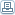# How You Should Use NPV to Evaluate Investment Real Estate

 By: James KobzeffNet present value is the present value of all future cash flows produced by a rental property less the amount of initial cash investment required to purchase the investment property.Net present value (NPV) considers the time value of money and therefore is a popular real estate investing rate of return. Let's say you require a 10% yield (rate of return) on your investment. Net present value (with consideration for the timing) reveals whether the cash flow produced by that property would give you a 10% rate of return.How it WorksLet's say that you require a 10% rate of return. This percentage then becomes the rate at which future cash flows are "discounted". Moreover, you plan to invest \$100,000. This is also known as initial investment and represents the down payment plus closing costs. Next, you estimate what the annual cash flows will be over the next (let's say five) years. Finally, you estimate your proceeds from a sale of the investment property in the (let's say fifth) year. Since the money you collect upon a sale is considered income, it's added to the cash flow derived in that same year.Here's how it looks:Discount Rate: 10.0%Initial Investment: \$100,000Cash Flow # 1 [estimated first year cash flow]Cash Flow # 2 [estimated second year cash flow]Cash Flow # 3 [estimated third year cash flow]Cash Flow # 4 [estimated fourth year cash flow]Cash Flow # 5 [estimated fifth year cash flow] + [sale proceeds]How to CalculateOkay, now to arrive at a net present value we must do the following.1. Discount all forecasted future cash flows at 10% to arrive at their present value (PV), remembering to add the forecasted sales proceeds to the cash flow in the final year. Let's say the present value for all five cash flows plus sale proceeds is \$100,000.2. Deduct the \$100,000 initial investment from the \$100,000 PV to determine the NPV. In this case, NPV would equal zero.What it MeansNet present value is a dollar amount (not a percentage rate) and will always result in one of the following amounts.1. Greater than zero - this means that the discounted value of the future cash flows is greater than the initial investment and thus you're getting a higher rate of return than you desired2. Equal to zero - this means that the discounted value of the future cash flows is exactly equal to your initial investment and thus you're getting the return you desired exactly3. Less than zero - this means that the discounted value of the future cash flows is less than the initial investment and thus you're getting a lower rate of return than you desiredAs stated earlier, net present value is commonly used in real estate investing by investors and analysts to evaluate investment real estate opportunities. It should not be used as the only factor to decide whether a rental property provides a good buying opportunity, but NPV does offer the investor a quick and easy way to determine whether a property might yield the investor's desired rate of return.You can make your own computations for net present value on Excel, with some real estate investment software programs, or with the help of a real estate professional who understands real estate investing.